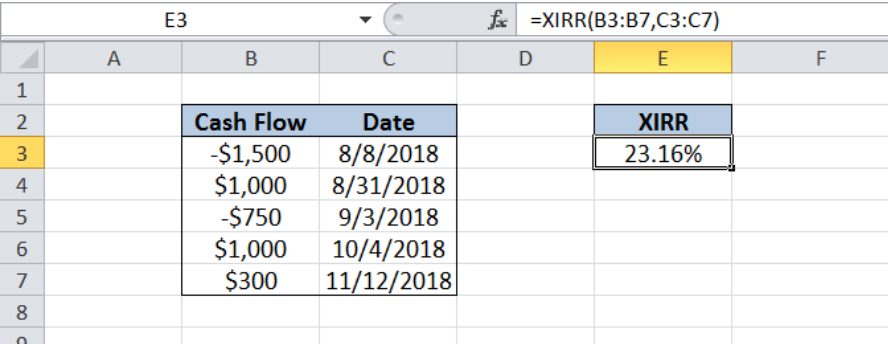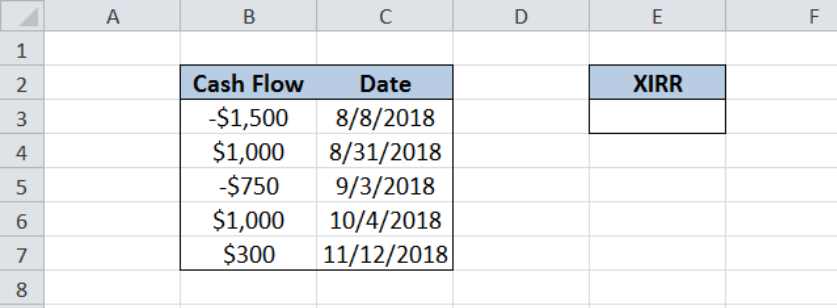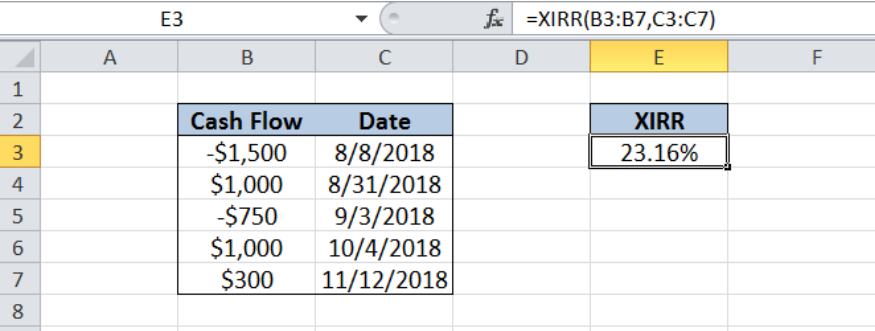Get instant live expert help with Excel or Google Sheets“My Excelchat expert helped me in less than 20 minutes, saving me what would have been 5 hours of work!”

#### Post your problem and you'll get expert help in seconds

Your message must be at least 40 characters
Our professional experts are available now. Your privacy is guaranteed.

# Excel XIRR Function

The XIRR function in Excel calculates the internal rate of return for scheduled but not necessarily periodic cash flows.  This tutorial will assist all levels of Excel users in calculating the internal rate of return for cash flows occurring at irregular intervals.Figure 1. Result of XIRR function

## Syntax of the XIRR Function

`=XIRR(values, dates, [guess])`

where

• Values – a series of cash flows with corresponding dates for schedule of payments. The series of values must contain at least one positive and one negative value.  Negative values indicate payments while positive values indicate income. All succeeding payments are discounted based on a 365-day year.
• Dates – corresponding schedule of payments for the cash flows.  Dates should be valid dates and should not be entered as text to avoid errors.
• GuessOptional; A number that we guess is close to the result of XIRR

## Setting up the Data

Our table consists of two columns: Cash Flow (column B) and Date (column C).  In cell E3, we calculate the internal rate of return using the XIRR function.Figure 2. Sample data for the XIRR formula

## Calculate internal rate of return using XIRR

In order to calculate the internal rate of return for the series of cash flows, we follow these steps:

Step 1. Select cell E3

Step 2. Enter the formula: `=XIRR(B3:B7,C3:C7)`

Step 3. Press Enter

The range for the cash flow is B3:B7 while the range for the dates is C3:C7.  As a result, the internal rate of return in E3 is 23.16%.Figure 3. Using the XIRR function to calculate internal rate of return

## Note

Excel calculates the internal rate of return through iteration up to the 0.000001% accuracy.  If the XIRR formula cannot find a result on the 100th try, the function returns a #NUM! Error.

Most of the time, the problem you will need to solve will be more complex than a simple application of a formula or function. If you want to save hours of research and frustration, try our live Excelchat service! Our Excel Experts are available 24/7 to answer any Excel question you may have. We guarantee a connection within 30 seconds and a customized solution within 20 minutes.

### Did this post not answer your question? Get a solution from connecting with the expert.Another blog reader asked this question today on Excelchat:## Subscribe to Excelchat.coAnother blog reader asked this question today on Excelchat: